# DAV Class 5 Maths Chapter 4 Worksheet 11 Solutions

The DAV Class 5 Maths Book Solutions Pdf and DAV Class 5 Maths Chapter 4 Worksheet 11 Solutions of Fractional Numbers offer comprehensive answers to textbook questions.

## DAV Class 5 Maths Ch 4 Worksheet 11 Solutions

Question 1.
Divide.
(a) $$\frac{1}{5}$$ ÷ 3
Solution: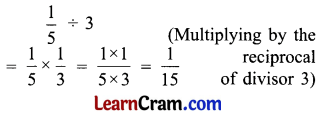(b) 2$$\frac{4}{5}$$ ÷ 6
Solution:(c) 2$$\frac{3}{5}$$ ÷ 4
Solution:(d) 18 ÷ $$\frac{3}{4}$$
Solution: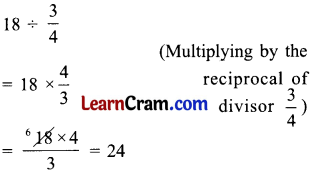(e) 20 ÷ 3$$\frac{1}{3}$$
Solution:(f) 100 ÷ 33$$\frac{1}{3}$$
Solution:(g) $$\frac{4}{5} \div \frac{7}{9}$$
Solution: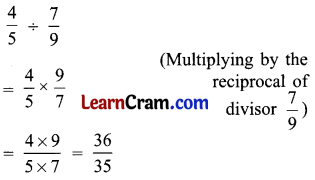(h) $$2 \frac{2}{3} \div 1 \frac{1}{6}$$
Solution:(i) $$10 \frac{1}{3} \div 4 \frac{1}{2}$$
Solution:(j) $$8 \frac{1}{4} \div 3 \frac{5}{8}$$
Solution:(k) $$2 \frac{1}{10} \div 1 \frac{2}{5}$$
Solution: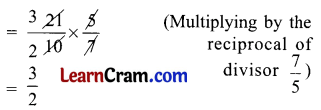(l) $$6 \frac{3}{5} \div 4 \frac{7}{12}$$
Solution: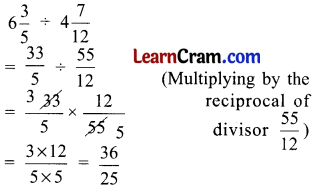DAV Class 5 Maths Chapter 4 Worksheet 11 Notes

I. Division of a fractional number by a whole number.

Example 1.
Divide $$\frac{3}{8}$$ by 2.
Solution:
Look at the figure.This represents $$\frac{3}{8}$$
Let us divide $$\frac{3}{8}$$ into 2 equal halves.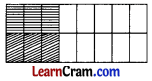This represents $$\frac{3}{16}$$
∴ $$\frac{3}{8}$$ ÷ 2 = $$\frac{3}{16}$$
In another manner
$$\frac{3}{8} \div 2=\frac{3}{8} \times \frac{1}{2}=\frac{3}{16}$$
So, dividing $$\frac{3}{8}$$ by 2 actually means multiplying $$\frac{3}{8}$$ with the reciprocal of 2 i.e., $$\frac{1}{2}$$.

Important Note: To divide first change the division sign to the multiplication sign and multiply by the reciprocal of the divisor.Example 2.
Divide 3$$\frac{1}{7}$$ by 3.
Solution: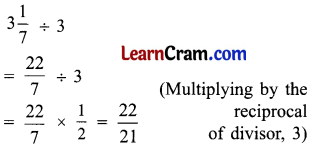II. Division of a whole number by a fractional number.

Example 3.
Divide 5 by $$\frac{2}{3}$$.
Solution: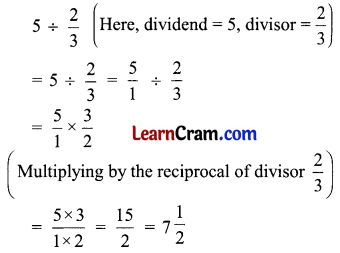III. Division of a fractional number by another fractional number.

Example 4.
Divide $$\frac{4}{3}$$ by $$\frac{7}{5}$$.
Solution:
$$\frac{4}{3} \div \frac{7}{5}$$
Here, dividend = $$\frac{4}{3}$$, divisor = $$\frac{7}{5}$$
= $$\frac{4}{3} \times \frac{5}{7}$$ (Multiplying by the reciprocal of divisor $$\frac{7}{5}$$)
= $$\frac{20}{21}$$